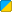# G.Lerp

 Function Syntax Lerp( Float frac, Float start, Float end ) Description: Performs a linear interpolation between start and end. The number returned is exactly "frac" of the way between start and end. Returns: Float Part of Library: Global Functions Realm:BBCode Link: [b][url=http://wiki.garrysmod.com/?title=G.Lerp]G.Lerp [img]http://wiki.garrysmod.com/favicon.ico[/img][/url][/b]

## Example

Description Lerps between the two numbers`print( Lerp( 0.25, 90, 0 ) );` 67.5

• frac is a number between 0 and 1. If frac is outside of this range it is automatically clamped.
• Because of the above, returned values will always be equal to or between the start/end values you gave.
• When frac is 0 the returned value is start
• When frac is 1 the returned value is end